## Example Questions

### Example Question #11 : Negative Numbers

x, y and z are negative numbers.

A

---

x + y + z

B

---

xyz

Quantity A is greater

The relationship cannot be determined

Quantity B is greater

The two quantities are equal

The relationship cannot be determined

Explanation:

Recognize the rules of negative numbers: if two negative numbers are multiplied, the result is positive. However if three negative numbers are multiplied, the result is negative. As such, we know B must be negative.

Since there are no restrictions on the values of x, y and z beyond being negative, lets check low values and high values: if every value was -1, multiplying the values would equal -1 while adding them would equal -3. However, if every value was -5, multiplying them would equal -125 while adding them would equal a mere -15. As such, we would need additional information to determine whether A or B would be greater.

### Example Question #71 : Integers

Simplify: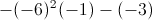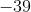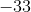Explanation: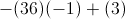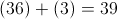Remember, the product of two negatives is positive. Also note that subtracting a negative is equivalent to adding its absolute value.

### Example Question #1 : How To Multiply Negative Numbers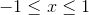and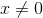Quantity AQuantity B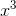Quantity B is greater.

The relationship cannot be determined from the information given.

The two quantities are equal.

Quantity A is greater.

The relationship cannot be determined from the information given.

Explanation:

Imagine two different scenarios when x equals either extreme: –1 or 1. If x equals –1, then x squared equals 1 and x cubed equals –1 (a negative times a negative times a negative is a negative), and thus Quantity A is greater. The other scenario is when x equals 1: x squared equals 1 and x cubed also equals 1. In this scenario, the two quantities are equal. Because both scenarios are possible, the relationship cannot be determined without more information.

### Example Question #1 : How To Multiply Negative Numbers

Ifandare both less than zero, which of the following is NOT possible?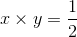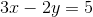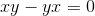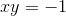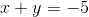Explanation:

This question tests your familiarity with the mathematical principles behind how negative numbers operate.is possible because two negative numbers added together will always equal a negative number.is possible because xy and -yx are inverses of each other, so they will combine to make 0.is possible because you don't know what the values of x and y are.  If y is sufficiently larger than x, then subtracting the negative number resulting from 2y (aka adding 2y) to the negative number 3x could be a positive number, including 5.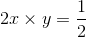is possible because a negative (2x) times a negative (y) will always be positive.

Which, of course, means thatis impossible, because a negative times a negative will never equal a negative.

Tired of practice problems?

Try live online GRE Math prep today.